# What Is the Difference Between PCA and LDA?

High dimensionality is one of the challenging problems machine learning engineers face when dealing with a dataset with a huge number of features and samples. Because of the large amount of information, not all contained in the data is useful for exploratory analysis and modeling. Some of these variables can be redundant, correlated, or not relevant at all. A popular way of solving this problem is by using dimensionality reduction algorithms – namely, principal component analysis (PCA) and linear discriminant analysis (LDA). The key idea is to reduce the volume of the dataset while preserving as much of the relevant data as possible.

In this tutorial, we are going to cover these two approaches, focusing on the main differences between them. At first sight, LDA and PCA have many aspects in common, but they are fundamentally different when looking at their assumptions. Then, we’ll learn how to perform both techniques in Python using the sk-learn library.

## What Is Principal Component Analysis?

Principal component analysis (PCA) is surely the most known and simple unsupervised dimensionality reduction method. By definition, it reduces the features into a smaller subset of orthogonal variables, called principal components – linear combinations of the original variables. The first component captures the largest variability of the data, while the second captures the second largest, and so on.

In essence, the main idea when applying PCA is to maximize the data's variability while reducing the dataset's dimensionality.

## What is Linear Discriminant Analysis?

Linear discriminant analysis (LDA) is a supervised machine learning and linear algebra approach for dimensionality reduction. It is commonly used for classification tasks since the class label is known.

Both LDA and PCA rely on linear transformations and aim to maximize the variance in a lower dimension. However, unlike PCA, LDA finds the linear discriminants in order to maximize the variance between the different categories while minimizing the variance within the class.

What’s key is that, where principal component analysis is an unsupervised technique, linear discriminant analysis takes into account information about the class labels as it is a supervised learning method. Moreover, it assumes that the data corresponding to a class follows a Gaussian distribution with a common variance and different means.

LDA is useful for other data science and machine learning tasks, like data visualization for example. Used this way, the technique makes a large dataset easier to understand by plotting its features onto 2 or 3 dimensions only.

## How To Perform PCA and LDA in Python?

As previously mentioned, principal component analysis and linear discriminant analysis share common aspects, but greatly differ in application. We’ll show you how to perform PCA and LDA in Python, using the sk-learn library, with a practical example.

### Importing the MNIST Dataset

To better understand what the differences between these two algorithms are, we’ll look at a practical example in Python. For this tutorial, we’ll utilize the well-known MNIST dataset, which provides grayscale images of handwritten digits.

The dataset, provided by sk-learn, contains 1,797 samples, sized 8 by 8 pixels. Our task is to classify an image into one of the 10 classes (that correspond to a digit between 0 and 9):

digits = load_digits()
df = pd.DataFrame(digits.data, columns=digits.feature_names)
df['target'] = digits.target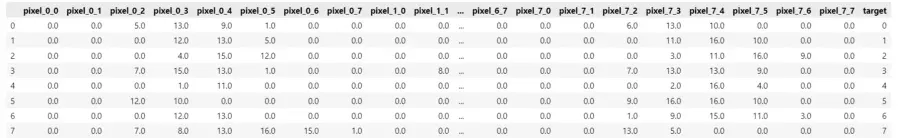The head() functions displays the first 8 rows of the dataset, thus giving us a brief overview of the dataset. Additionally, there are 64 feature columns that correspond to the pixels of each sample image and the true outcome of the target.

### Standardizing the Numerical Features

Our goal with this tutorial is to extract information from this high-dimensional dataset using PCA and LDA. We are going to use the already implemented classes of sk-learn to show the differences between the two algorithms.

However, before we can move on to implementing PCA and LDA, we need to standardize the numerical features:

X = digits.data
X = StandardScaler().fit_transform(X)
y = digits.target

This ensures they work with data on the same scale.

## How to Perform PCA in Python?

Now that we’ve prepared our dataset, it’s time to see how principal component analysis works in Python.

### Choosing the Number of Components

First, we need to choose the number of principal components to select. To do so, fix a threshold of explainable variance – typically 80%.

Now, the easier way to select the number of components is by creating a data frame where the cumulative explainable variance corresponds to a certain quantity. We apply a filter on the newly-created frame, based on our fixed threshold, and select the first row that is equal or greater than 80%:

pca = PCA().fit(X)
np_cum = np.cumsum(pca.explained_variance_ratio_)
df_cum = pd.DataFrame({'Component':[i for i in range(1,np_cum.shape+1)],'cum_explained_variance_ratio':np_cum})
filter_df = df_cum[df_cum.cum_explained_variance_ratio>=0.8]
component = int(filter_df.Component.iloc)
cum_var = float(filter_df.cum_explained_variance_ratio.iloc)
print(f'{component} Components capture {round(cum_var,4)*100}% of variability of the data')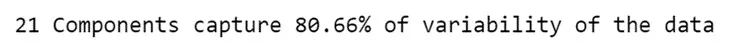As a result, we observe 21 principal components that explain at least 80% of variance of the data.

We can get the same information by examining a line chart that represents how the cumulative explainable variance increases as soon as the number of components grow:

threshold = 0.8
fig = plt.figure(figsize=(14,8))
plt.plot(df_cum.cum_explained_variance_ratio)
plt.xlabel(‘Number of Components’)
plt.ylabel(‘Cumulative Explained Variance’)
plt.axhline(y=threshold, color = ‘r’, linestyle = ‘—')
plt.axvline(x=component, color = ‘r’, linestyle = ‘—')
plt.show()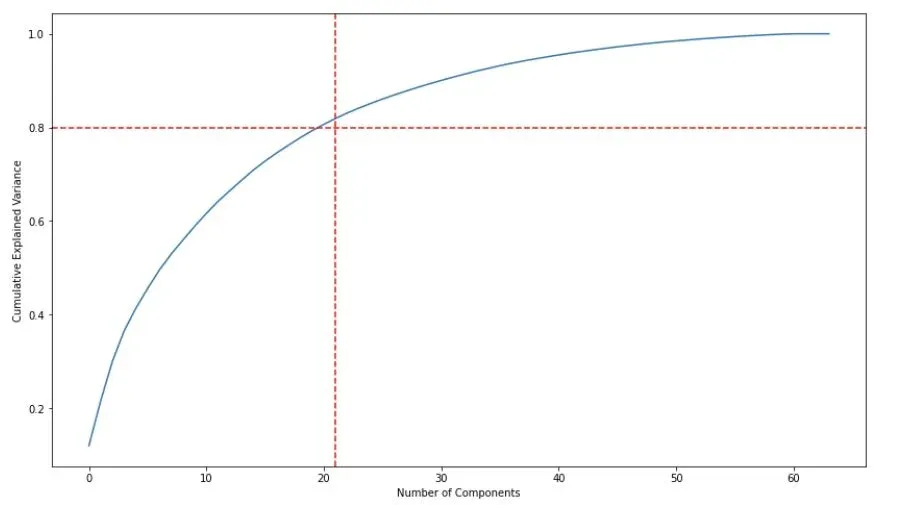By looking at the plot, we see that most of the variance is explained with 21 components, same as the results of the filter.

### Performing Dimensionality Reduction with PCA

Let’s reduce the dimensionality of the dataset using the principal component analysis class:

X = StandardScaler().fit_transform(X)
pca = PCA(n_components=21)
X_pca = pca.fit_transform(X)


The first thing we need to check is how much data variance each principal component explains through a bar chart:

fig = plt.figure(figsize=(14,8))
plt.bar(range(1,22),pca.explained_variance_ratio_,)
plt.ylabel('Explained variance ratio')
plt.xlabel('Principal components')
plt.xlim([0.5,22])
plt.xticks(range(1,22))
plt.show()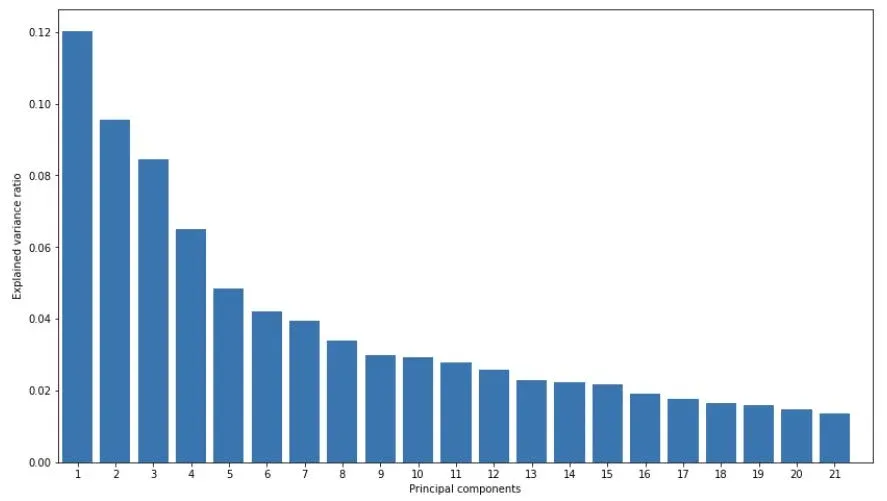The first component alone explains 12% of the total variability, while the second explains 9%. The percentages decrease exponentially as the number of components increase.

Let’s plot the first two components that contribute the most variance:

fig = plt.figure(figsize=(14,8))
plt.scatter(X_pca[:, 0], X_pca[:, 1],
c=digits.target, edgecolor='none', alpha=0.5,
cmap=plt.cm.get_cmap('nipy_spectral', 10))
plt.xlabel('Principal component 1')
plt.ylabel('Principal component 2')
plt.colorbar()
plt.show()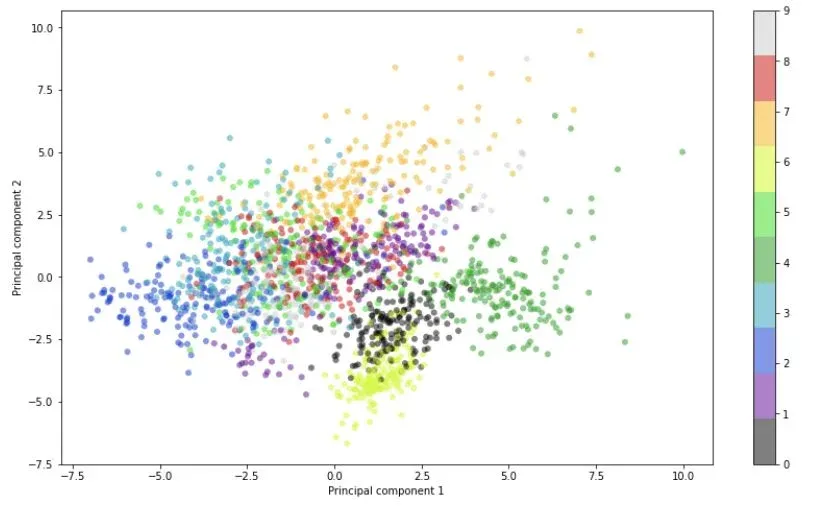In this scatter plot, each point corresponds to the projection of an image in a lower-dimensional space. Furthermore, we can distinguish some marked clusters and overlaps between different digits. For example, clusters 2 and 3 (marked in dark and light blue respectively) have a similar shape – we can reasonably say that they are overlapping.

To have a better view, let’s add the third component to our visualization:

import matplotlib.cm as cm
fig = plt.figure(figsize=(18,10))
ax = plt.axes(projection='3d')
p = ax.scatter3D(X_pca[:, 0], X_pca[:, 1],X_pca[:, 2],
c=digits.target, edgecolor='none', alpha=0.5,
cmap=plt.cm.get_cmap('nipy_spectral', 10))
ax.set_xlabel('Principal component 1')
ax.set_ylabel('Principal component 2')
ax.set_zlabel('Principal component 3')
plt.colorbar(p)
plt.show()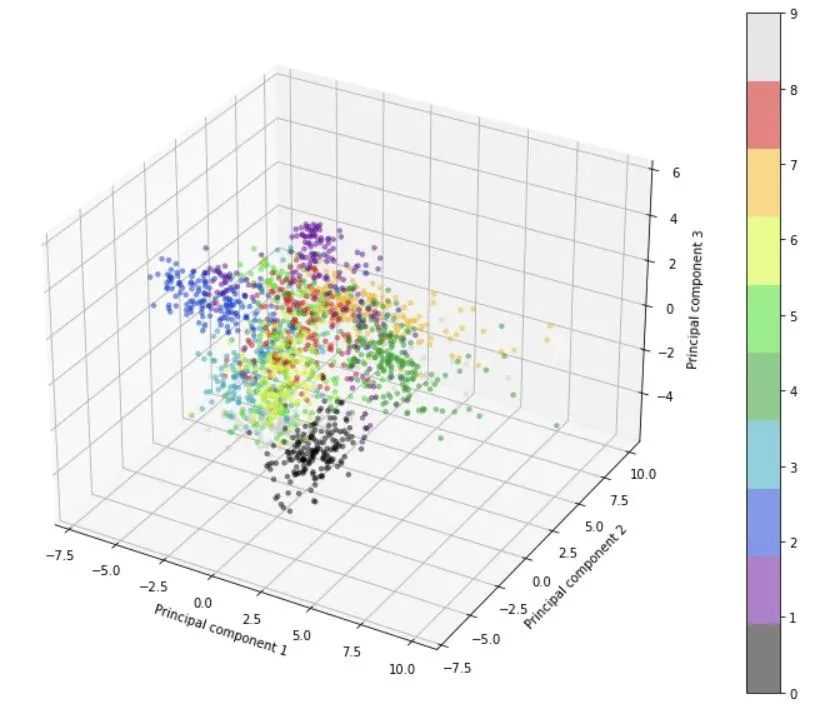This creates a higher-dimensional plot that better shows us the positioning of our clusters and individual data points. Though not entirely visible on the 3D plot, the data is separated much better, because we’ve added a third component. For example, now clusters 2 and 3 aren’t overlapping at all – something that was not visible on the 2D representation.

## How to Perform LDA in Python?

Let’s now try to apply linear discriminant analysis to our Python example and compare its results with principal component analysis:

lda = LDA(n_components=21)
X_lda = lda.fit_transform(X,y)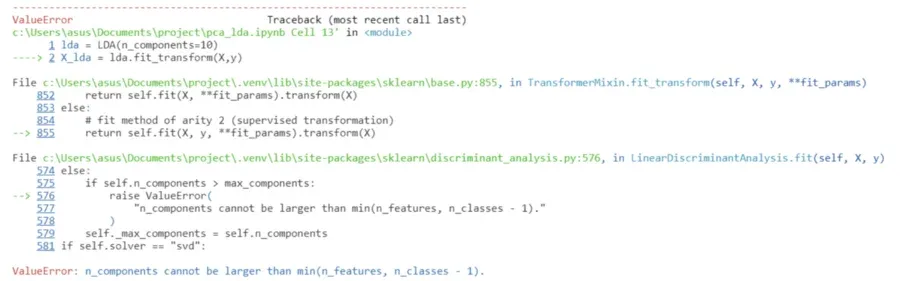From what we can see, Python has returned an error.

As it turns out, we can’t use the same number of components as with our PCA example since there are constraints when working in a lower-dimensional space:

$$k \leq \text{min} (\# \text{features}, \# \text{classes} - 1)$$

In this case, the categories (the number of digits) are less than the number of features and have more weight to decide k. We have digits ranging from 0 to 9, or 10 overall. Using the formula to subtract one of classes, we arrive at 9.

### Choosing the Number of Components

We can follow the same procedure as with PCA to choose the number of components:

lda = LDA()
X_lda = lda.fit_transform(X,y)
np_cum_lda = np.cumsum(lda.explained_variance_ratio_)
df_cum_lda = pd.DataFrame({'Component':[i for i in range(1,np_cum_lda.shape+1)],'explained_variance_ratio':lda.explained_variance_ratio_,'cum_explained_variance_ratio':np_cum_lda})
print(df_cum_lda)
filter = df_cum_lda[df_cum_lda.cum_explained_variance_ratio>0.8].iloc
component = int(filter.Component)
cum_var = float(filter.cum_explained_variance_ratio)
print(f"\n{component} Discriminant Components explain {round(cum_var,4)*100}% of variability between CLASSES")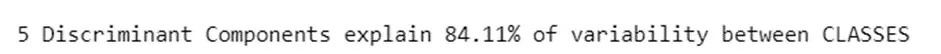While the principle component analysis needed 21 components to explain at least 80% of variability on the data, linear discriminant analysis does the same but with fewer components.

However, the difference between PCA and LDA here is that the latter aims to maximize the variability between different categories, instead of the entire data variance!

Let’s visualize this with a line chart in Python again to gain a better understanding of what LDA does:

threshold = 0.8
fig = plt.figure(figsize=(14,8))
plt.plot(np.cumsum(lda.explained_variance_ratio_))
plt.xlabel('Number of components')
plt.ylabel('Cumulative Explained Variance');
plt.axhline(y=threshold, color = 'r', linestyle = '--')
plt.axvline(x=component, color = 'r', linestyle = '--')
plt.show()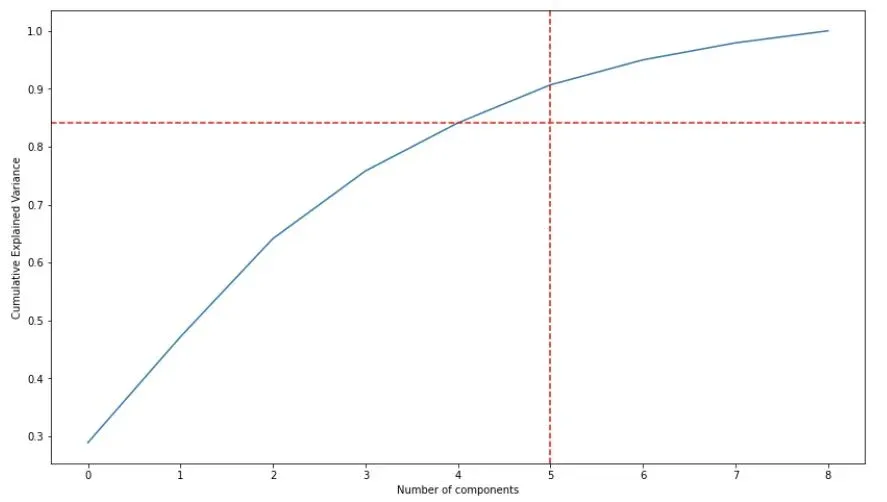It seems the optimal number of components in our LDA example is 5, so we’ll keep only those.

Now, let’s visualize the contribution of each chosen discriminant component:

lda = LDA(n_components=5)
X_lda = lda.fit_transform(X,y)
fig = plt.figure(figsize=(14,8))
plt.bar(range(1,6),lda.explained_variance_ratio_,)
plt.ylabel('Explained variance ratio')
plt.xlabel('Discriminant components')
plt.xlim([0.5,6])
plt.xticks(range(1,6))
plt.show()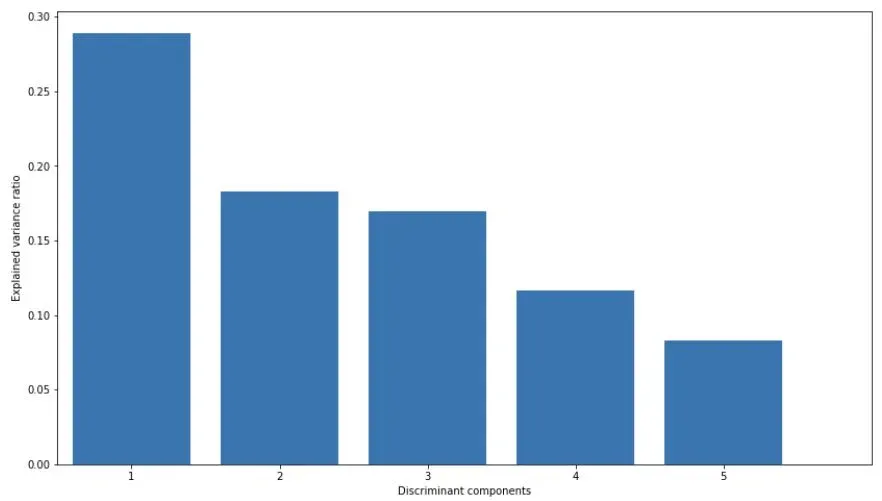Our first component preserves approximately 30% of the variability between categories, while the second holds less than 20%, and the third – only 17%. Similarly to PCA, the variance decreases with each new component.

Let’s plot our first two using a scatter plot again:

fig = plt.figure(figsize=(14,8))
plt.scatter(X_lda[:, 0], X_lda[:, 1],
c=digits.target, edgecolor='none', alpha=0.5,
cmap=plt.cm.get_cmap('nipy_spectral', 10))
plt.xlabel('Discriminant component 1')
plt.ylabel('Discriminant component 2')
plt.colorbar()
plt.show()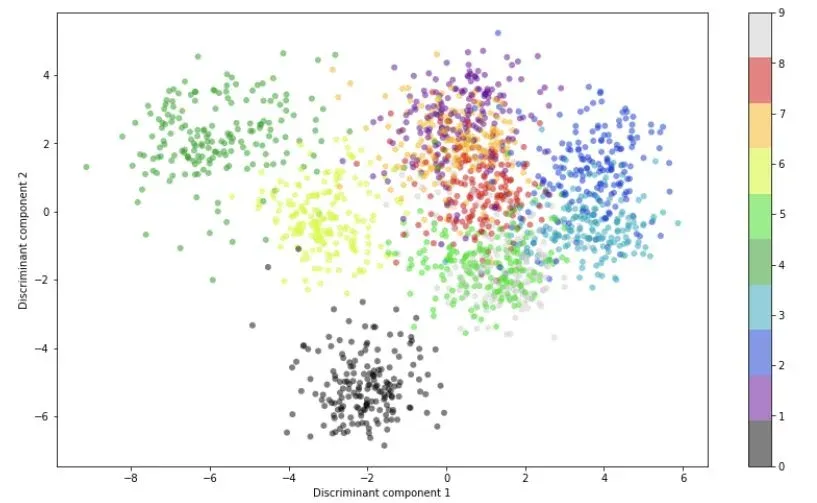This time around, we observe separate clusters representing a specific handwritten digit, i.e. they are more distinguishable than in our principal component analysis graph.

The results are motivated by the main LDA principles to maximize the space between categories and minimize the distance between points of the same class. In the meantime, PCA works on a different scale – it aims to maximize the data’s variability while reducing the dataset’s dimensionality.

Moreover, linear discriminant analysis allows to use fewer components than PCA because of the constraint we showed previously, thus it can exploit the knowledge of the class labels. For these reasons, LDA performs better when dealing with a multi-class problem.

We can also visualize the first three components using a 3D scatter plot:

fig = plt.figure(figsize=(18,10))
ax = plt.axes(projection='3d')
p=ax.scatter3D(X_lda[:, 0], X_lda[:, 1],X_lda[:, 2],
c=digits.target, edgecolor='none', alpha=0.5,
cmap=plt.cm.get_cmap('nipy_spectral', 10))
ax.set_xlabel('Discriminant component 1')
ax.set_ylabel('Discriminant component 2')
ax.set_zlabel('Discriminant component 3')
plt.colorbar(p)
plt.show()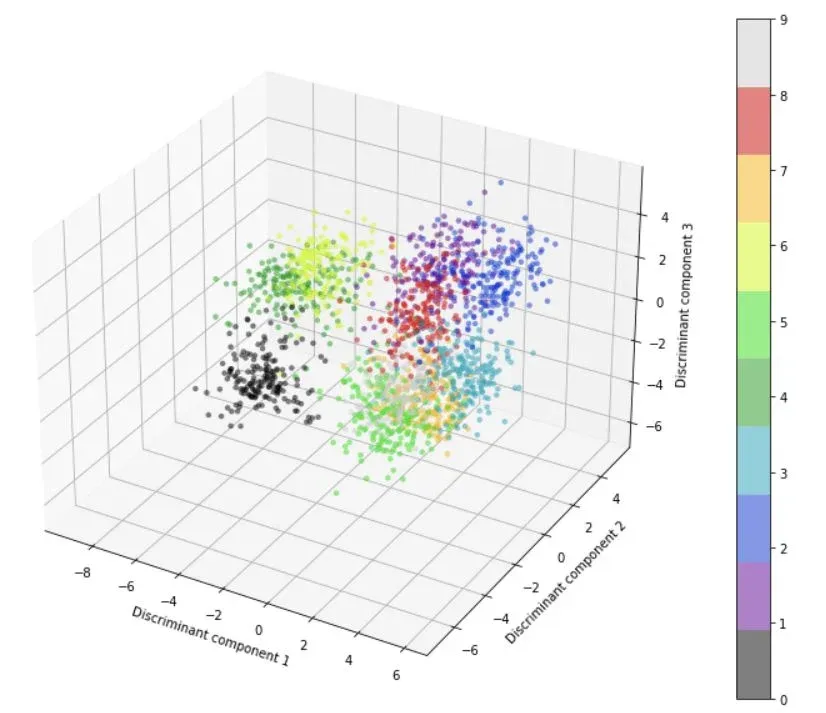Et voilà! This last gorgeous representation that allows us to extract additional insights about our dataset. As we can see, the cluster representing the digit 0 is the most separated and easily distinguishable among the others.

In contrast, our three-dimensional PCA plot seems to hold some information, but is less readable because all the categories overlap. At the same time, the cluster of 0s in the linear discriminant analysis graph seems the more evident with respect to the other digits as it’s found with the first three discriminant components.

We can safely conclude that PCA and LDA can be definitely used together to interpret the data. As a matter of fact, LDA seems to work better with this specific dataset, but it can be doesn’t hurt to apply both approaches in order to gain a better understanding of the dataset.

## PCA vs LDA: Next Steps

Principal component analysis and linear discriminant analysis constitute the first step toward dimensionality reduction for building better machine learning models. If you want to improve your knowledge of these methods and other linear algebra aspects used in machine learning, the Linear Algebra and Feature Selection course is a great place to start!

Be sure to check out the full 365 Data Science Program, which offers self-paced courses by renowned industry experts on topics ranging from Mathematics and Statistics fundamentals to advanced subjects such as Machine Learning and Neural Networks. If you want to see how the training works, sign up for free with the link below.

Learn data science with industry expertsStart your career